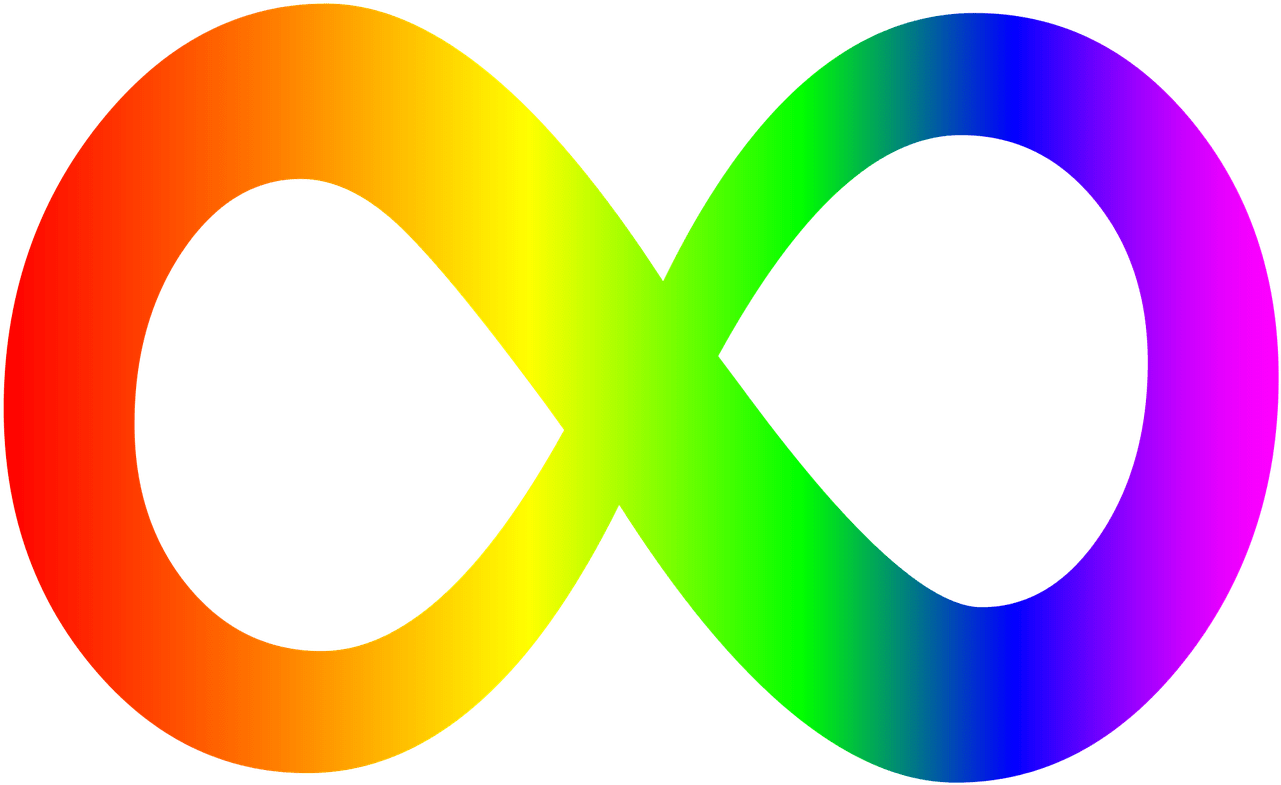Language: English | España | РусскийShare image:

Keywords: Infinity symbol PNG size: 1281x785px, Infinity symbol PNG images, PNG image: Infinity symbol PNG, free PNG image, Infinity symbol
Image category: Infinity symbol
Format: PNG image with alpha (transparent)
Resolution: 1281x785
Size: 82 kb

# Infinity symbol PNG image with transparent background | symbol_infinity_PNG18.png

This image has format transparent PNG with resolution 1281x785.

# Infinity symbol

Infinity is that which is boundless, endless, or larger than any natural number. It is often denoted by the infinity symbol {displaystyle infty }infty .

Since the time of the ancient Greeks, the philosophical nature of infinity was the subject of many discussions among philosophers. In the 17th century, with the introduction of the infinity symbol and the infinitesimal calculus, mathematicians began to work with infinite series and what some mathematicians (including l'Hôpital and Bernoulli) regarded as infinitely small quantities, but infinity continued to be associated with endless processes. As mathematicians struggled with the foundation of calculus, it remained unclear whether infinity could be considered as a number or magnitude and, if so, how this could be done. At the end of the 19th century, Georg Cantor enlarged the mathematical study of infinity by studying infinite sets and infinite numbers, showing that they can be of various sizes. For example, if a line is viewed as the set of all of its points, their infinite number (i.e., the cardinality of the line) is larger than the number of integers. In this usage, infinity is a mathematical concept, and infinite mathematical objects can be studied, manipulated, and used just like any other mathematical object.

The mathematical concept of infinity refines and extends the old philosophical concept, in particular by introducing infinitely many different sizes of infinite sets. Among the axioms of Zermelo–Fraenkel set theory, on which most of modern mathematics can be developed, is the axiom of infinity, which guarantees the existence of infinite sets. The mathematical concept of infinity and the manipulation of infinite sets are used everywhere in mathematics, even in areas such as combinatorics that may seem to have nothing to do with them. For example, Wiles's proof of Fermat's Last Theorem implicitly relies on the existence of very large infinite sets for solving a long-standing problem that is stated in terms of elementary arithmetic.

In physics and cosmology, whether the Universe is infinite is an open question.

The infinity symbol ({displaystyle infty }infty ) is a mathematical symbol representing the concept of infinity. This symbol is also called a lemniscate, after the lemniscate curves of a similar shape studied in algebraic geometry, or "lazy eight", in the terminology of livestock branding.

This symbol was first used mathematically by John Wallis in the 17th century, although it has a longer history of other uses. In mathematics, it often refers to infinite processes (potential infinity) rather than infinite values (actual infinity). It has other related technical meanings, such as the use of long-lasting paper in bookbinding, and has been used for its symbolic value of the infinite in modern mysticism and literature. It is a common element of graphic design, for instance in corporate logos as well as in older designs such as the Métis flag.

Both the infinity symbol itself and several variations of the symbol are available in various character encodings.